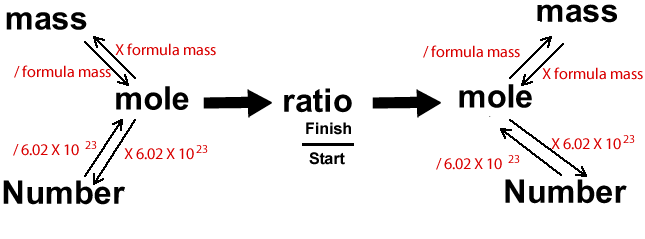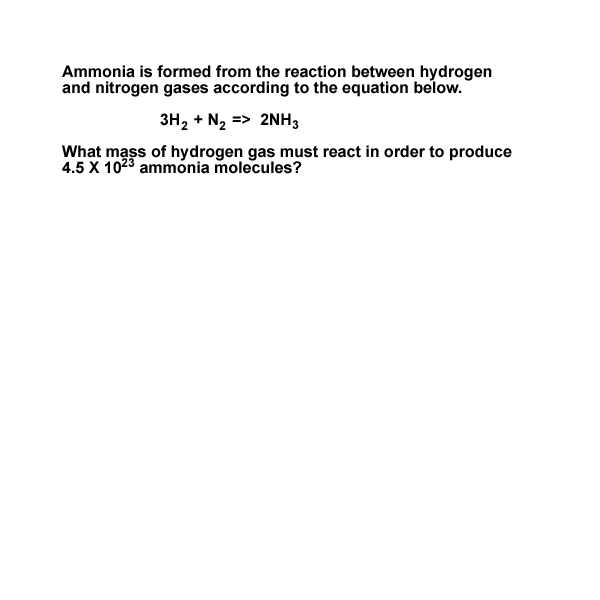Calculations with the stoichiometry diagram continued Calculations in chemistry can be a little overwhelming. It can sometimes help to visualise the problem through diagrams. Below is a diagram that I find very useful indeed.Ammonia is formed from the rection between hydrogen and nitrogen gases according to the reaction below. 3H2 + N2 => 2NH3 What mass of hydrogen gas must react in order to produce 4.5 X 1023 molecules of ammonia? We are given the number of ammonia molecules and we are asked for the mass of hydrogen gas that reacts. We must therefore start at "number", because we know the number of ammonia molecules and go to "mass", on the other side of the diagram, to find the mass of hydrogen.Continue